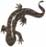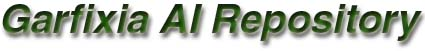A genetic algorithm can be really easy to implement. I made it a little harder for myself by creating a generic library in stead of a domain specific algorithm for some specific problem. My aim is to build tools to be used again.

What was implemented:
• Three classes and an interface. The interface (CGeneticGenome) should be implemented by a custom class describing the problem space. It contains all domain specific code of some problem. The first class, CGeneticModel, represents the genetic algorithm. It may be customized in a few ways, and excuted. This model uses a single population, of the class CGeneticPopulation, which is populated at initialization time. After that, the individuals of the population, the genomes, are changed and moved only, but not destroyed and created. This should make a lot of difference in execution time.
• The model is able to handle both fixed length and variable length genomes.
• The interface makes it possible to use any representation you want for the problem at hand. The interface serves as an interface (indeed) between the application and the algorithm.
• Parent selection types (used to select parents from the previous generation to serve as parents for the next generation): Selection by rank, Roulette Wheel, and Tournament.
• Crossover types: fixed length one point, fixed length two points, fixed length multiple, variable length one point.
• Mutation types: change a gene, swap two genes.
What was not implemented:

Actually, this implementation is generic, but very limited. The possibilities of extending and modifying a genetic algorithm are large. I made a consideration between studying long on this subject to gain deeper insight in its intricacies, and moving on to other subjects and I chose the latter, because its more important to me now to gain a wide understanding of available AI techniques. I do believe that my architecture may be extended without to much hardship.

Remarks
• Genetic Algorithms can be addictive. The drug is to make more and more adjustments to the algorithm to make it find the solution in the fastest possible way. The problem for me was not to lose the generality of the architecture, in order to optimize the test problem I choose (the n-Queens problem).
• I found that testing is very important here, since the algorithm can almost always find a solution sooner or later, even though you completely fucked up some of the code. At one time I removed a bug and noticed that the algorithm become slower. That was really annoying.
• Since the algorithm is tweakable in so many ways, it itself is a candidate for optimization through a Genetic Algorithm. I leave that as an exercise to the reader :*)
Sample use

This sample solves the n-Queens problem. As you can see, the domain specific class takes up most of the space.

```class CQueenGenome: public CGeneticGenome
{
protected:
int n;
int *y;

public:
CQueenGenome(int new_n)
{
n = new_n;
y = new int[n];
}

~CQueenGenome(){ delete[] y; }

/// create a new genome, no randomization is required
virtual CGeneticGenome *NewGenome(){ return new CQueenGenome(n); }

/// create default (random) values for the genes
virtual void Initialize()
{
for (int i=0; i<n; i++) MutateGene(i);
}

/// set the length (i.e. the number of genes)
virtual void SetLength(int NewLength){}

/// return the length
virtual int GetLength(void){ return n; }

/// copy gene at position Pos to Child at pos ChildPos
virtual void CopyGene(int Pos, CGeneticGenome *Child, int ChildPos)
{
((CQueenGenome *)Child)->y[ChildPos] = y[Pos];
}

/// is this genome equal to Genome
virtual bool Equals(CGeneticGenome *Genome)
{
for (int i=0; i<n; i++)
{
if (y[i] != ((CQueenGenome *)Genome)->y[i]) return false;
}
return true;
}

/// store a random value in the gene
virtual void MutateGene(int GeneIndex)
{
int old_y, new_y;

old_y = y[GeneIndex];

do
{
new_y = CMath::GetRandomInt(0, n-1);
}
while (new_y == old_y);

y[GeneIndex] = new_y;
}

/// calculate and return the fitness of this genome
virtual void CalculateFitness(void)
{
int piece, piece_x, piece_y, pos_x, pos_y;
Fitness = 0.0f;

for(piece=0; piece<n; piece++)
{
piece_x = piece;
piece_y = y[piece];

// horizontal right
for(pos_x = piece_x+1, pos_y = piece_y; pos_x < n; pos_x++)
{
if (y[pos_x] == pos_y){ Fitness--; }
}
// horizontal left
for(pos_x = piece_x-1, pos_y = piece_y; pos_x >= 0; pos_x--)
{
if (y[pos_x] == pos_y){ Fitness--; }
}
// right up
for(pos_x = piece_x+1, pos_y = piece_y+1;
(pos_y < n) && (pos_x < n); pos_y++, pos_x++)
{
if (y[pos_x] == pos_y){ Fitness--; }
}
// right down
for(pos_x = piece_x+1, pos_y = piece_y-1;
(pos_y >= 0) && (pos_x < n); pos_y--, pos_x++)
{
if (y[pos_x] == pos_y){ Fitness--; }
}
// left up
for(pos_x = piece_x-1, pos_y = piece_y+1;
(pos_y < n) && (pos_x >= 0); pos_y++, pos_x--)
{
if (y[pos_x] == pos_y){ Fitness--; }
}
// left down
for(pos_x = piece_x-1, pos_y = piece_y-1;
(pos_y >= 0) && (pos_x >= 0); pos_y--, pos_x--)
{
if (y[pos_x] == pos_y){ Fitness--; }
}
}

Fitness = 1.0f + (Fitness / (2 * n));

if (Fitness < 0.0) Fitness = 0.0f;
}

/// is the genome fit enough to stop evolving?
virtual bool IsFitEnough(void)
{
return (Fitness == 1.0f);
}

virtual const CString ToString(void) const
{
CString String;

for (int i=0; i<n; i++)
{
String += y[i];
String += ' ';
}
return String;
}
};

int main(int argc, char* argv[])
{
// genetic algorithms
CQueenGenome QueenGenome(100);
CGeneticModel GeneticModel(&QueenGenome);

// this part is optional, it changes some settings of the algorithm
// for educational purposes, the default values are set here, just to
// show how it can be done
GeneticModel.ClearParentSelectionTypes();
GeneticModel.ClearCrossoverTypes();
GeneticModel.ClearMutationTypes();
GeneticModel.SetPopulationSize(100, 10, 10, -1);
GeneticModel.SetMutationRate(1.0f);
GeneticModel.Initialize();

// evolve the model until it found a fit enough genome
GeneticModel.Evolve();

// print out the genome's value
CGeneticGenome *FittestGenome = GeneticModel.GetFittestGenome();
printf("Genome %s\n", FittestGenome->ToString().GetBuffer());
}
```